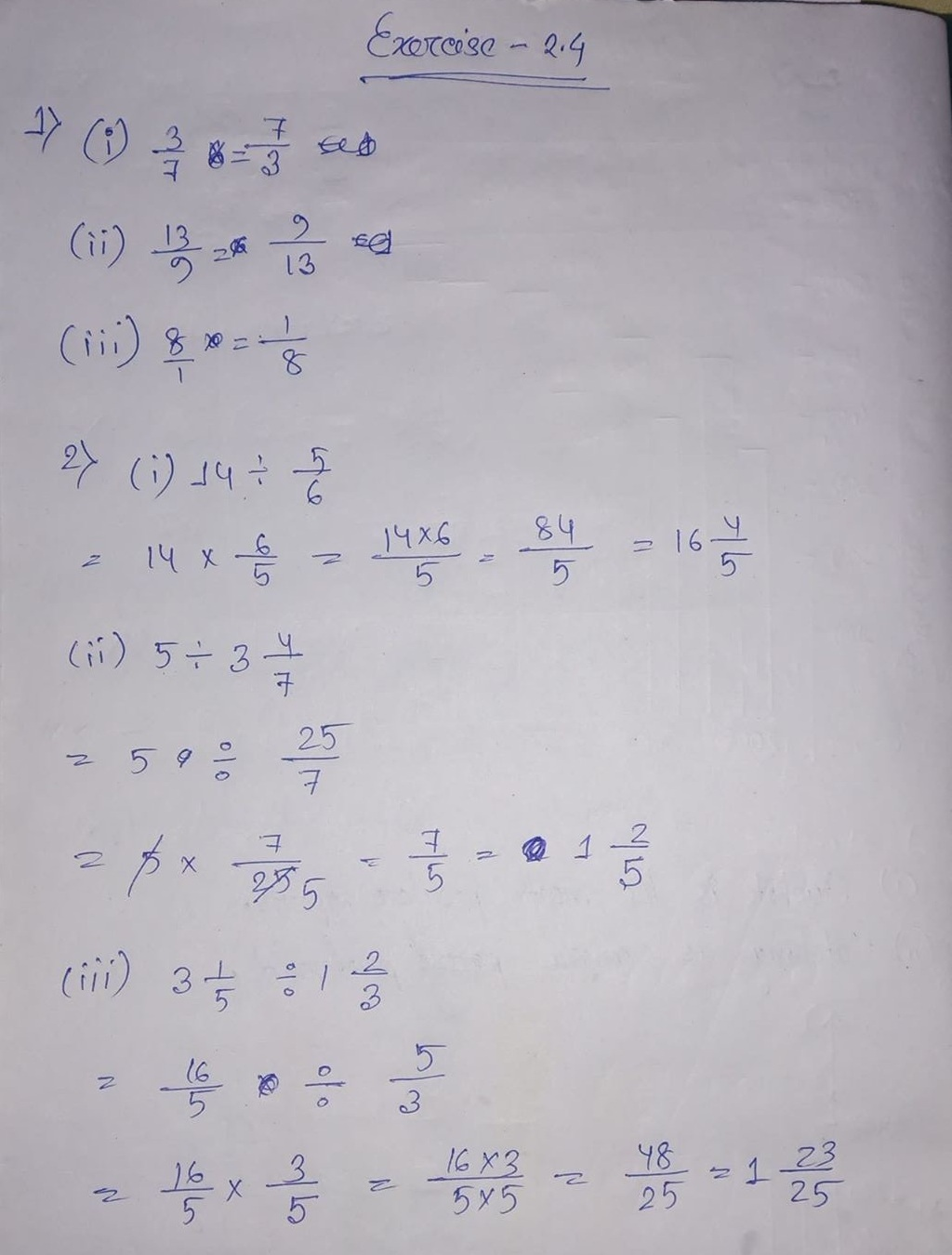# ML Aggarwal CBSE Solutions Class 7 Math Second Chapter Fractions and Decimals Exercise 2.4

### ML Aggarwal CBSE Solutions Class 7 Math 2nd Chapter Fractions and Decimals Exercise 2.4

Solution:(3) How many pieces each 5 1/6 metres long can be cut from a cloth 77 1/2 metres long?

(4) By what number should 4 7/8 be multiplied to get 87 3/4?

Solution:(5) In a hostel’s mess, each student gets 1/3 litre of milk everyday. If the total consumption of the milk is 57 2/3 litres per day. how many students are there in the hostel?

(6) The cost of 5 1/4 kg of apples is Rs. 336. What is the rate of apples per kg?

Solution:(7) The length of a rectangular plot of area 68 3/4 sq. m is 12 1/2 m, find its width.

(8) If the cost of 5 1/2 kg of sugar is Rs. 206 1/4, then find the cost of 8 1/4 kg of sugar.

Solution:(9) Renu completed 2/3 part of her homework in 2 hours. How much part of her homework had she completed in 1 1/4 hours?

Solution:Updated: December 6, 2019 — 1:18 pm